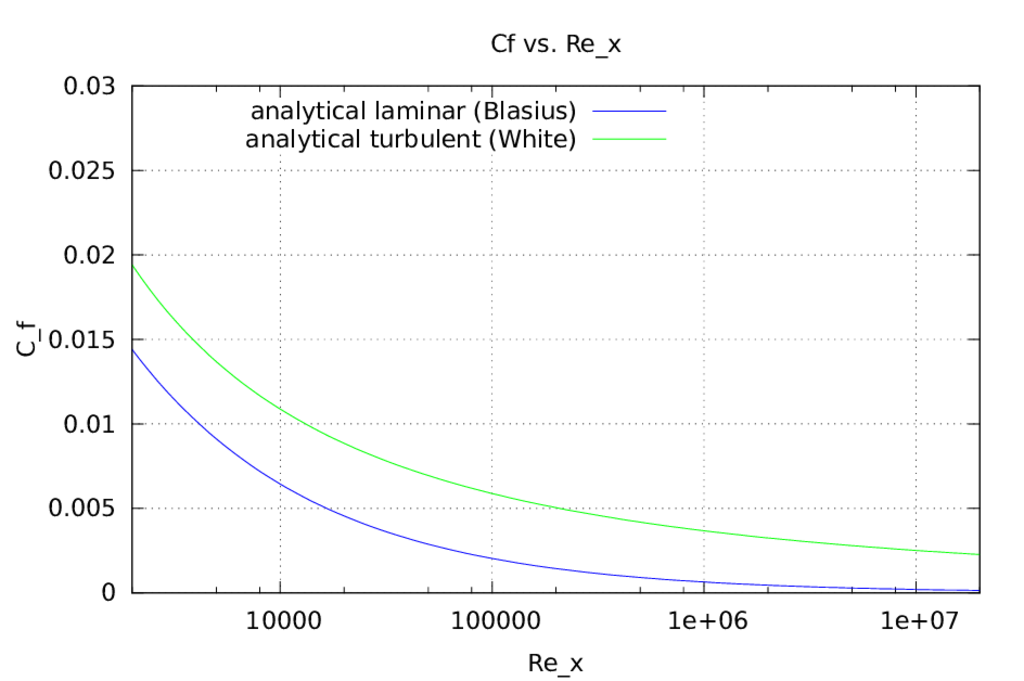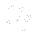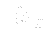This is an automatically generated documentation by LaTeX2HTML utility. In case of any issue, please, contact us at info@cfdsupport.com.

## Friction Coefficient vs. Reynolds-X

• Friction coefficientversus Reynolds based on x coordinateat the flat plate.
• Equations () show experimental correlation of various measurements.
• For laminar fluid flows at the flat plate the Blasius formula is used.
• For turbulent fluid flows at the flat plate the White formula is used.(25.1)

where:(25.2)Figure: Friction coefficientversus Reynolds based on x coordinateat the flat plate. Equationsshow experimental correlation of various measurements. For laminar fluid flows at the flat plate the Blasius formula is used. For turbulent fluid flows at the flat plate the White formula is used.